### IMO Shortlist 1973 problem 17

Kvaliteta:
Avg: 0.0
Težina:
Avg: 0.0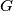$G$ is a set of non-constant functions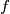$f$. Each$f$ is defined on the real line and has the form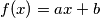$f(x)=ax+b$ for some real$a,b$. If$f$ and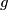$g$ are in$G$, then so is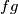$fg$, where$fg$ is defined by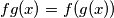$fg(x)=f(g(x))$. If$f$ is in$G$, then so is the inverse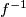$f^{-1}$. If$f(x)=ax+b$, then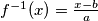$f^{-1}(x)= \frac{x-b}{a}$. Every$f$ in$G$ has a fixed point (in other words we can find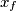$x_f$ such that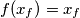$f(x_f)=x_f$. Prove that all the functions in$G$ have a common fixed point.
Source: Međunarodna matematička olimpijada, shortlist 1973